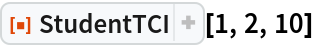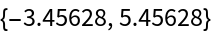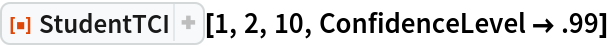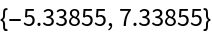#Function Repository Resource:

# StudentTCI

Estimate the confidence interval based on Student's t-distribution

Contributed by: Wolfram Research
 ResourceFunction["StudentTCI"][μ,σ,df] gives a confidence interval based on Student's t-distribution with df degrees of freedom.

## Details and Options

ResourceFunction["StudentTCI"][μ,σ,df] gives a confidence interval {min,max} centered at μ based on Student's t-distribution with location parameter μ, scale parameter σ and df degrees of freedom.
ResourceFunction["StudentTCI"] is the confidence interval for a population mean based on a sample mean μ and standard error σ estimated from a sample of size df + 1.
The following option can be given:
 ConfidenceLevel 0.95 probability associated with a confidence interval

## Examples

### Basic Examples (1)

A 95% confidence interval based on Student's t-distribution with 10 degrees of freedom:

 In:=Out=### Options (1)

#### ConfidenceLevel (1)

A 99% confidence interval:

 In:=Out=## Requirements

Wolfram Language 11.3 (March 2018) or above